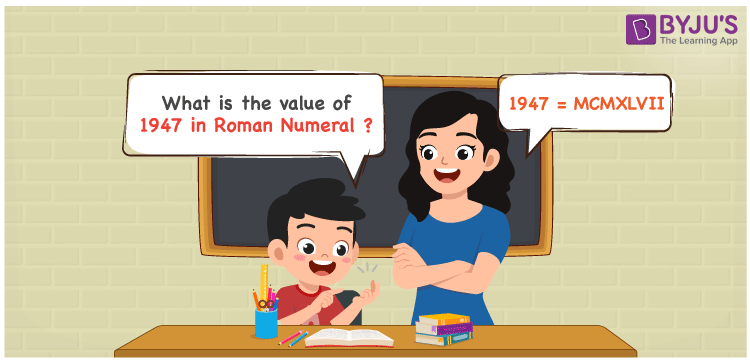# 1947 in Roman Numerals

1947 in Roman Numerals is written as MCMXLVII. The letters used by the ancient Romans to denote numbers are known as Roman Numerals. For instance, I, IV, VIII, and XL represent 1, 4, 8, and 40 respectively. In this article, we will discuss how to convert 1947 into Roman Numerals using simple rules in an efficient manner.

 Number Roman Numeral 1947 MCMXLVII

## How to Write 1947 in Roman Numerals?The below method is used to write 1947 in Roman Numerals

• First, break 1947 into the least expandable form
• 1947 = 1000 + (1000 – 100) + (50 – 10) + 5 + 1 + 1
• Write their respective Roman Numerals and add/subtract them
• 1947 = M + (M – C) + (L – X) + V + I + I = MCMXLVII
• Hence, the value of 1947 in Roman Numerals is MCMXLVII

## Video Lesson on Roman Numerals## Frequently Asked Questions on 1947 in Roman Numerals

### How do you write 1947 in Roman Numerals?

We write 1947 in Roman Numerals as MCMXLVII.

### What is the value of 3000 – 1947? Express the answer in Roman Numerals.

3000 – 1947 = 1053. To express 1053 in Roman Numerals, we write 1053 into the simplest form i.e., 1053 = 1000 + 50 + 1 + 1 + 1 = M + L + I + I + I = MLIII. Therefore the value of 3000 – 1947 in Roman Numerals is MLIII.

### Calculate the value of (1900 + 200) – 1947 in Roman Numerals.

(1900 + 200) – 1947 = 153. Now, 153 = 100 + 50 + 1 + 1 + 1 = C + L + I + I + I = CLIII. Therefore the value of (1900 + 200) – 1947 in Roman Numerals is CLIII.